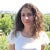# Convolutional Neural Networks for Image Classification

In deep learning, convolutional neural networks, also know as ConvNets or CNNs, are a class of deep neural networks that have proven to be powerful in the domain of computer vision. They are used in various fields such facial recognition, medical imaging, self-driving cars etc.

This article explains how convolutional neural networks works and gives an example of how it can be applied for image classification using TensorFlow and Keras. The article is divided into the following two main parts:

• Architecture of Convolutional Neural Networks
• Building a basic CNN Model Using TensorFlow and Keras

# Architecture of Convolutional Neural Networks

Convolutional Neural Networks are special kinds…

# A Brief Introduction to Deep Learning

Deep learning is a subset of machine learning that uses multi-layered neural networks to extract useful features from raw data. It has widely advanced the state-of-the-art in various fields such as computer vision, speech recognition and natural language processing.

This article aims to give an introduction to deep learning by covering the following points:

• What is the difference between machine learning and deep learning?
• How do deep learning networks work?
• Applications of deep learning networks

# Machine Learning vs. Deep Learning

Deep learning is a class of machine learning; thus, it is considered a machine learning technique with special capabilities. As seen in figure 1, machine…

# Machine Learning to Predict the Survivals of Titanic

The titanic survival prediction project is a well known project for beginners in the field of data science. It covers all the basics of data cleaning, data exploration, data visualization and machine learning.

In this article, I will explain how I approached this challenge to achieve an accuracy of 0.8 and scored in the top 9% of Kaggle's Titanic competition.

# 1. Necessary Libraries

The libraries/packages that are used in this projects are:

• pandas: a library used for data analysis and processing.
• matplotlib: a library used for data visualization. It is a plotting library for Python.
• seaborn: a library built on top of matplotlib…## Kheirie Elhariri

Data Scientist I AI Enthusiast | Passionate about Education